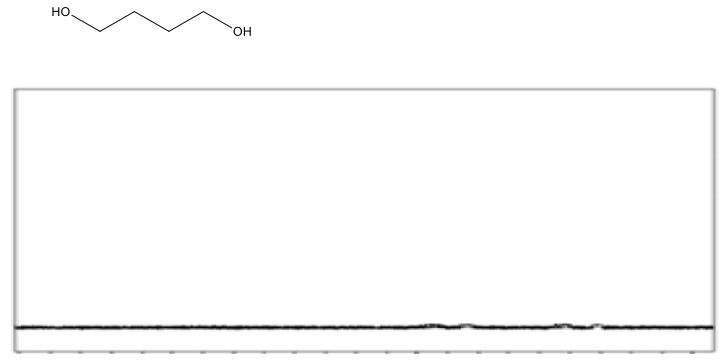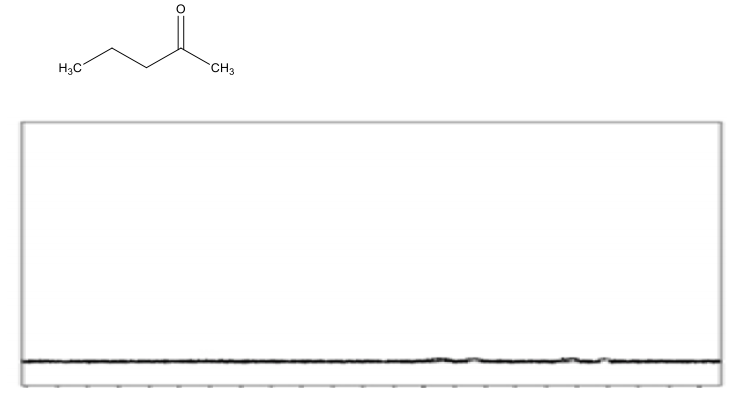Clutch Prep is now a part of Pearson
Ch. 15 - Analytical Techniques: IR, NMR, Mass SpectWorksheetSee all chapters

# NMR Practice

See all sections
Sections
Purpose of Analytical Techniques
Infrared Spectroscopy
Infrared Spectroscopy Table
IR Spect: Drawing Spectra
IR Spect: Extra Practice
NMR Spectroscopy
1H NMR: Number of Signals
1H NMR: Q-Test
1H NMR: E/Z Diastereoisomerism
H NMR Table
1H NMR: Spin-Splitting (N + 1) Rule
1H NMR: Spin-Splitting Simple Tree Diagrams
1H NMR: Spin-Splitting Complex Tree Diagrams
1H NMR: Spin-Splitting Patterns
NMR Integration
NMR Practice
Carbon NMR
Structure Determination without Mass Spect
Mass Spectrometry
Mass Spect: Fragmentation
Mass Spect: Isotopes

Practice: Draw the approximate positions that the following compound might show in its 1H NMR absorptions?Practice: Draw the approximate positions that the following compound might show in its 1H NMR absorptions?